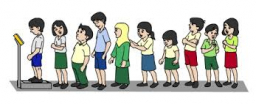# Attended 47663

Three times more boys than girls attended the student meeting. When 8 boys and 8 girls left early, five times more boys than girls stayed at the meeting. How many boys and how many girls were at the game?

a =  48
b =  16

### Step-by-step explanation:

a=3b
a-8=5(b-8)

a=3·b
a-8=5·(b-8)

a-3b = 0
a-5b = -32

Row 2 - Row 1 → Row 2
a-3b = 0
-2b = -32

b = -32/-2 = 16
a = 0+3b = 0+3 · 16 = 48

a = 48
b = 16

Our linear equations calculator calculates it.Did you find an error or inaccuracy? Feel free to write us. Thank you!

Tips for related online calculators
Do you have a linear equation or system of equations and looking for its solution? Or do you have a quadratic equation?